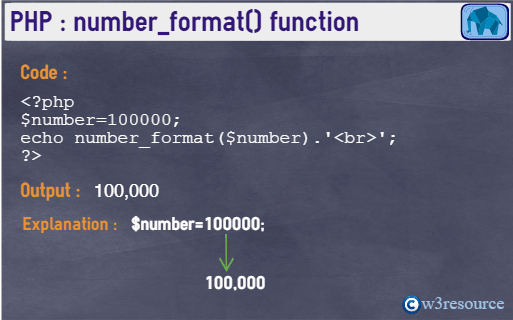# PHP: number_format() function

## Description

The number_format() function is used to format a number (Floating number) with grouped thousands.

Version:

(PHP 4 and above)

Syntax:

`number_format(number, decimals, dec_point, thousands_sep) `

Parameter:

Name Description Required /
Optional
Type
number The input number. Required Float
decimals Refer to the number of decimal points. Optional Integer
dec_point Refers the separator of decimal points. Optional String
thousands_sep Refers the thousands separator. Optional String

Note: The function accepts one, two, or four parameters (not three).

Return value:

A formatted version of the number.

Value Type: String

Pictorial PresentationExample:

``````<?php
\$number=100000;
echo number_format(\$number).'<br>';
echo number_format(\$number, 2).'<br>';
echo number_format(\$number, 3).'<br>';
echo number_format(\$number, 2, ',', '.');
?>``````

Output:

```100,000
100,000.00
100,000.000
100.000,00 ```

View the example in the browser

PHP Function Reference

Previous: nl2br
Next: ord

﻿

## PHP: Tips of the Day

echo and print: echo and print are language constructs, not functions. This means that they don't require parentheses around the argument like a function does (although one can always add parentheses around almost any PHP expression and thus echo("test") won't do any harm either). They output the string representation of a variable, constant, or expression. They can't be used to print arrays or objects.

Assign the string Joel to the variable \$name

Example:

```\$name = "Peter";
```

Output the value of \$name using echo & print

```<?php
\$name = "Peter";
echo \$name;
echo "\n";
print \$name;
?>
```

Output:

```Peter
Peter
```

Parentheses are not required, but can be used

```<?php
\$name = "Owen";
echo(\$name);
echo "\n";
print(\$name);
?>
```

Output:

```Owen
Owen
```

Using multiple parameters (only echo)

```<?php
\$name = "Jhon";
echo \$name, "Edward";
?>
```

Output:

```JhonEdward
```
```<?php
\$name = "Jhon";
echo \$name, " ", "Edward" ;
?>
```

Output:

```Jhon Edward
```

print, unlike echo, is an expression (it returns 1), and thus can be used in more places:

```<?php
print("Hello") && print(" ") && print("World");
?>
```

Output:

```World11
```

The above is equivalent to:

```<?php
print ("Hello" && (print (" " && print "World")));
?>
```

Output:

```World11
```